# Unequal-split Wilkinsons - the rest of the story

Click here to go to our main page on Wilkinson power splitters

Originally posted November 2008. This topic is so novel and important to microwave engineers that we actually considered whether it should be first published in the IEEE transactions. But that would delay the release of the material by six or eight months, to a far more limited audience than we have, what were we thinking? The world has been waiting 43 years for this topic to be further explored, and that's long enough. Hopefully the next IEEE author that discusses unequal-split Wilkinsons will reference our material, or they will hear from us on this page. So when the time comes, be sure to credit Editor, T., and Kouzoujian, J., affiliated with Microwaves101 and Matrix Test Equipment respectively, for developing the following material...

The original unequal-split Wilkinson article is entitled Split Tee Power Divider, by Parad and Moynihan, published in IEEE Transactions on Microwave Theory and Techniques in January, 1965. This article has been referenced in many subsequent works, including Harlan Howe's Stripline Circuit Design and Pozar's Microwave Engineering. In the article, Parad and Moynihan develop a singular set of equations for two unequal splitters. The simpler one is shown below (the second one adds an input transformer). For now, we will reanalyze the simpler one here.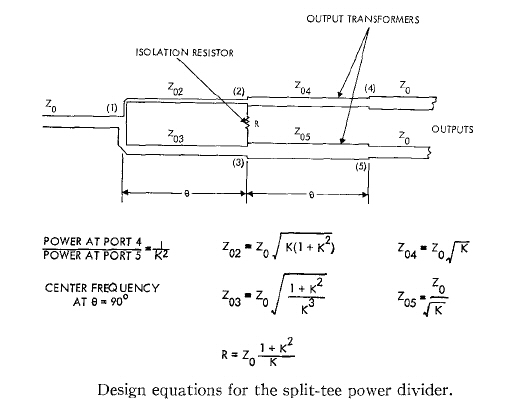We created our own nomenclature for the problem, which is provided in the figure below: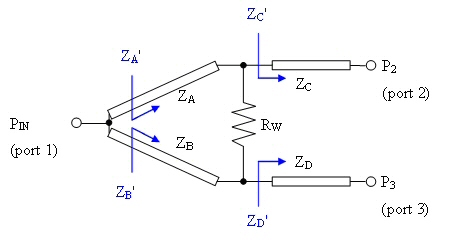Referring to our figure, the article states that the impedances ZC' and ZD' are equal to: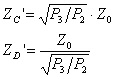These equations are based on an assumption that is not fully explained in the paper. If anyone can explain it to us, you will win a Microwaves101 pocketknife! The four line impedances can then be uniquely solved to agree with the solution that Parad and Moynihan provided. We rearranged their equations slightly but ours give the same result: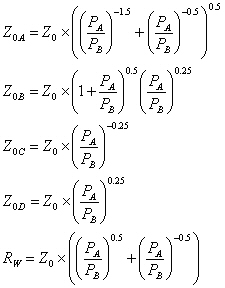Update June 2011: Igor offers the following explanation for how Parad and Moynihan came to this singular solution:

The ZC' and ZD' are set such that their geometric mean is equal to Z0.

ZC'=(P3/P2)^0.5*Z0

ZD'=(P3/P2)^-0.5*Z0

(ZC'*ZD')^0.5=Z0

This minimizes the impedance transformation ratios for a given power split and thus maximizes the bandwidt
h.

Thanks for that! The knife is in the mail...

Note: there is some confusion on this page about the definition of the power split. The way Parad and Moynihan defined it, the ratio of power delivered to port 2 to power delivered to port 3 is 1/K^2. Later, we might define this differently, so pay attention... we'll try to clean this all up one day!

The above equations are used in the Microwaves101 unequal-split Wilkinson on-line calculator, and have been used by thousands of engineers for over 40 years.

Taking a fresh look at the problem, we have found just three equations that must be satisfied for the four unknown impedances, which reveals that there is a degree of freedom that has been ignored for the past 43 years. The solution that Parad and Moynihan chose provides good bandwidth, but bandwidth could perhaps be traded for more realizable impedances, and design engineers should make that choice themselves.
The equations that are incontrovertible are based on three criteria that must be met. The impedances of the two arms have to combine in parallel to provide the system impedance Z0. In our figure, these impedances are denoted ZA' and ZB'. Then: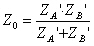(Equation 1)

Because the voltage at the input node is the same looking into each arm, the power split is proportional to the current split, which will be inversely proportional to the impedances ZA' and ZB' looking into the arms: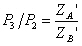(Equation 2A)

Therefore: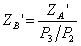(Equation 2B)

Substituting (2B) into (1) and solving for ZA' as well as ZB' we get: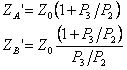(Equation 3)

Noting that ZA' and ZB' are functions of the two stage transformers ZA cascaded with ZC, and ZB cascaded with ZD, we see that: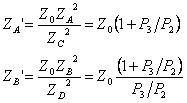(Equation 4A)

Which can be rearranged to: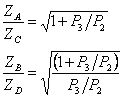(Equation 4B)

The voltage across the isolation resistor has to be zero, when power is applied from the common port. In order for this to happen, the impedance ratio must be maintained through the first legs of the splitter (ZA and ZB). Therefore: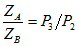(Equation 5)

From (4B) and (5) we now have three equations and four unknowns. We chose ZA as the independent variable and solved for ZB, ZC and ZD: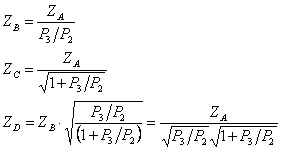(Equation 6)

Finally, we need to calculate the load resistor. This can be done easily by noting that in the odd mode, power incident into port 2 must see impedance matched to Z0 and power incident into port 3 must also see impedance matched to Z0 (see figure).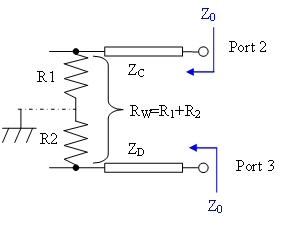Therefore: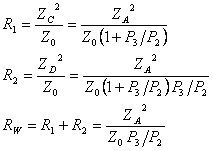(Equation 7)

With (6) and (7) our solution to the unequal split Wilkinson is complete. Now we can look at the impedance values when we choose different values of ZA. Let's look at a divider that splits two thirds energy to port 2, and one third to port 3 (P3/P2=0.5, see table below). Parad and Moynihan's results are ZA=51.5 ohms (shown in bold). Here the impedance for ZB is very high (103 ohms). By moving to ZA=40 ohms, the impedance range of the four lines is now 32.7 to 80 ohms, which may be more realizable in microstrip applications.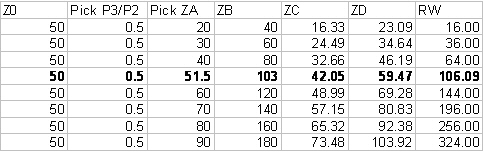What does this do to the frequency response? Below is a model of the splitter in ADS. We used this to look at the effects of varying ZA but maintaining a fixed power split. The equations in the "VAR" block are valid versions of the solution we have just provided. In this case, "ratio" is the ratio of power at port 3 to power at port 2, so "ratio=0.5" means that port 2 is favored with twice the power as port 3.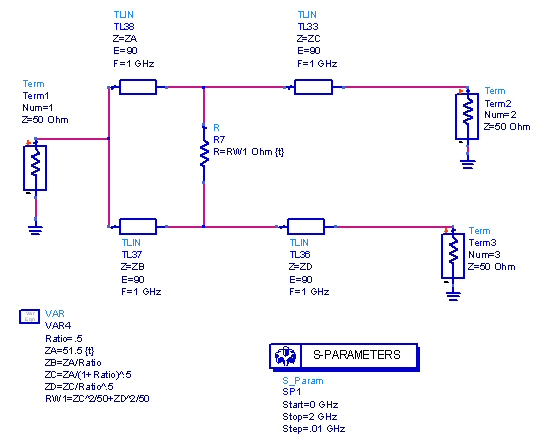Let's look at the response as ZA is varied. The center column of the figure below represents Parad's and Moynihan's solution, for a power divider where P3/P2 is 0.5 (ZA=51.5 ohms). Beside it are plots of the same power split but with PA=40 ohms, and PA=60 ohms. The markers shows that the power split is maintained. Click on an image to zoom in on it.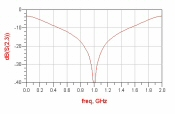Isolation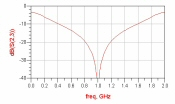Isolation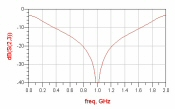Isolation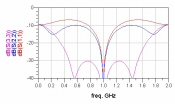Match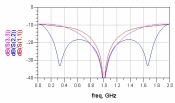Match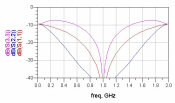Match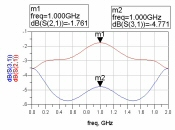Transmission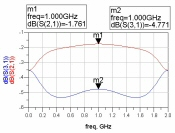Transmission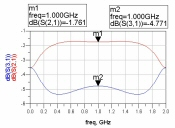Transmission ZA=40 ohms ZA=51.5 ohms ZA=60 ohms

Before we get much further, we want to point out one thing. For equations for solving the line impedances of an unequal splitter, you'd expect that for P3/P2 =x and P3/P2=1/x, you should have the same solution, only "flipped", right? If you use Parad and Moynihan's equations, this is what happens. For other solutions, this is something to watch out for (including the one below).

### Maximally flat transformer applied to unequal-split Wilkinson

Incorporating the maximally-flat impedance transformation to determine the section impedances provide for superior input match compared to Parad and Moynihan's solution. The exact solution to maximally-flat impedance transformer is not easy to solve, except in the case where the transformer only has two sections.

Unfortunately the dog ate this part of our homework, but when it is completed the unequal/max flat divider design will appear on this page. Stay tuned!

Author : Unknown Editor